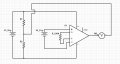# AD 620 Instrumentation Amplifier simple connection diagram

#### Mihran

Joined Nov 3, 2017
3
Hello.

I am new in pcb design except of student years.

I am trying to connect AD620 Instrumentation amplifier.According to AD620 datasheet, Gain = [(49.4 kOm / R(gain) ) + 1]. Thus, in case of R(gain) = 14.7 kOm, the Gain of the AD620 should be 4.36.

PARTS PROPERTIES

R1 = R2 = 21.7 kOm
R (gain) = 14.7 kOm
V1 = 5V
V2 = I change the values from controller's GUI

MEASUREMENTS RESULTS (M2 Voltmeter).

V2 = 0.2 V - - - - - M2 = -2.115V
V2 = 0.5 V - - - - - M2 = -2.115V
V2 = 0.8 V - - - - - M2 = -2.099V
V2 = 1 V - - - - - M2 = -2.012V

As you can see there are incorrect results.

Please, help me to understand what is incorrect.

Thanks.

#### crutschow

Joined Mar 14, 2008
26,064
V2 and VREF are floating.
They both has to be referenced to the virtual ground voltage (junction of R1 and R2).

Since VREF has a 10kΩ impedance it must be connected to a low impedance virtual ground, which can be made by connecting an op amp follower circuit to the junction of R1 and R2.

Easier is to use two power supplies as shown in the data sheet.

#### Mihran

Joined Nov 3, 2017
3

The V1 and V2 are generated from the same controller(configurable from GUI) and have the same ground.

There is no circuit following to OP AMP. I just wont to see gained signal on the multimeter screen.

#### GopherT

Joined Nov 23, 2012
8,012

The V1 and V2 are generated from the same controller(configurable from GUI) and have the same ground.

There is no circuit following to OP AMP. I just wont to see gained signal on the multimeter screen.

That is the point, you cannot be successful with a simple multimeter, your InAmp is amplifying the output signal, but your reference needs to be current amplified (with an opAmp) to make sure you have an accurate output. If you don't need an accurate output and can accept a 1 to 10% error (or so), then you can connect the multimeter to the ref and output pins. Before doing that, you cneed to connect V2and Vref to the center node of a voltage divider made With two 1k resistors and connecting them to 0 and Vsupply